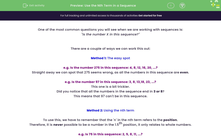# Use the Nth Term in a Sequence

In this worksheet, students will use the nth term to confirm if a number does or does not appear in a given sequence.Key stage:  KS 4

Year:  GCSE

GCSE Subjects:   Maths

GCSE Boards:   AQA, Eduqas, Pearson Edexcel, OCR,

Curriculum topic:   Algebra

Curriculum subtopic:   Sequences

Difficulty level:#### Worksheet Overview

One of the most common questions you will see when we are working with sequences is:

"Is the number X in this sequence?"

There are a couple of ways we can work this out:

Method 1: The easy spot

e.g. Is the number 275 in this sequence: 4, 8, 12, 16, 20, ...?

Straight away we can spot that 275 seems wrong, as all the numbers in this sequence are even.

e.g. Is the number 97 in this sequence: 3, 8, 13,18, 23, ...?

This one is a bit trickier.

Did you notice that all the numbers in the sequence end in 3 or 8?

This means that 97 can't be in this sequence.

Method 2: Using the nth term

To use this, we have to remember that the 'n' in the nth term refers to the position.

Therefore, it is never possible to be a number in the 1.5th position, it only relates to whole numbers.

e.g. Is 75 in this sequence: 2, 5, 8, 11, ...?

Step 1: Find the nth term of the sequence.

For this sequence, our nth term would be 3n - 1.

Step 2: Make this nth term equal to the number you are looking for and then solve the equation.

3n - 1 = 75

3n = 76

n = 25.3333

Because n isn't a whole number, this means that 75 cannot be in this sequence.

e.g. Is 224 in this sequence: 8, 14, 20, 26, ...?

Step 1: Find the nth term of the sequence.

For this sequence, our nth term would be 6n + 2.

Step 2: Make this nth term equal to the number you are looking for and then solve the equation.

6n + 2 = 224

6n = 222

n = 37

Because n is a whole number, 224 is in this sequence.

In this activity, we will use the nth term to confirm if a number does or does not appear in a given sequence. If we can, we will use the 'easy spot' method first in order to save time and effort.

### What is EdPlace?

We're your National Curriculum aligned online education content provider helping each child succeed in English, maths and science from year 1 to GCSE. With an EdPlace account you’ll be able to track and measure progress, helping each child achieve their best. We build confidence and attainment by personalising each child’s learning at a level that suits them.

Get started